Courses

# JEE(MAIN) Mathematics Mock Test - 4

## 30 Questions MCQ Test JEE Main Mock Test Series 2020 & Previous Year Papers | JEE(MAIN) Mathematics Mock Test - 4

Description
This mock test of JEE(MAIN) Mathematics Mock Test - 4 for JEE helps you for every JEE entrance exam. This contains 30 Multiple Choice Questions for JEE JEE(MAIN) Mathematics Mock Test - 4 (mcq) to study with solutions a complete question bank. The solved questions answers in this JEE(MAIN) Mathematics Mock Test - 4 quiz give you a good mix of easy questions and tough questions. JEE students definitely take this JEE(MAIN) Mathematics Mock Test - 4 exercise for a better result in the exam. You can find other JEE(MAIN) Mathematics Mock Test - 4 extra questions, long questions & short questions for JEE on EduRev as well by searching above.
QUESTION: 1

Solution:
QUESTION: 2

Solution:
QUESTION: 3

### Let f(x) be a function satisfying f ′(x) = f (x) with f(0) = 1 and g(x) be a function that satisfies f(x) + g(x) = x2, then value of integral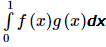is equal to

Solution:
QUESTION: 4

If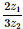is a purely imaginary number, then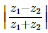is equal to

Solution:
QUESTION: 5

If f (x) = cos [π2] x + cos [− π2] x where [x] is the step function, then

Solution:
QUESTION: 6

The sum of the focal distances from any point on the ellipse 9x2 + 16y2 = 144 is

Solution:
QUESTION: 7

In the following question, a Statement of Assertion (A) is given followed by a corresponding Reason (R) just below it. Read the Statements carefully and mark the correct answer-
Assertion(A): f (x) = log x3 and g (x) = 3 log x are equal.
Reason(R) : Two functions f and g are said to be equal if their domains, ranges are equal and f (x) = g (x)∀ x in the domain .

Solution:
QUESTION: 8

Determinant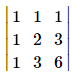is not equal to

Solution:
QUESTION: 9

If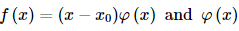is continuous at x = x0 , then f ′ (x0) is equal to

Solution:
QUESTION: 10

Solution of the differential equation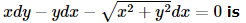Solution:
QUESTION: 11

In the following question, a Statement-1 is given followed by a corresponding Statement-2 just below it. Read the statements carefully and mark the correct answer-
Tangents are drawn from the point (17,7) to the circle x2+y2=169.
Statement-1:
The tangents are mutually perpendicular.
Statement-2:
The locus of the points from which mutually perpendicular tangents can be drawn to the given circle is x2+y2=338.

Solution:
QUESTION: 12

If z2 = -i, then z is equal o

Solution:
QUESTION: 13

The sum of the digits in the unit place of all the numbers formed with the help of 3,4,5,6 taken all at a time is

Solution:
If the unit place is ‘3’ then remaining three places can be filled in 3! ways.
Thus ‘3’ appears in unit place in 3! times.
Similarly each digit appear in unit place 3! times.
So,  sum of digits in unit place = 3!(3 + 4 + 5 + 6) = 18 * 6 = 108
QUESTION: 14

If θ + Φ = π/3 then sin θ . sinΦ has a maximum value at θ =

Solution:

Here, y = sin θ · sinΦ = sinθ · sin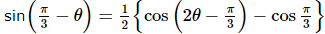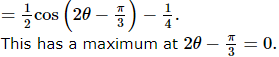QUESTION: 15

An unbiased coin is tossed to get 2 points for turning up a head and one point for the tail. If three unbiased coins are tossed simultaneously, then the probability of getting a total of odd number of points is

Solution:
QUESTION: 16
The probability of A = probability of B = probability of C = 1/4, P(A∩B) = P(C∩B) = 0 and P(A∩C) = 1/8, then P(A∪B∪C) is equal to:
Solution:
QUESTION: 17

If the equations x2+ ba + a = 0 and x2 + ax + b = 0 have a common root, then a + b =

Solution:
QUESTION: 18

If a, b, c, d, e, f are in A.P., then e - c is equal to

Solution:
QUESTION: 19

If a line passes through points (4,3) and (2,λ) and perpendicular to y=2x+3, then λ=

Solution:
QUESTION: 20

The median of a set of 9 distinct observations is 20.5. If each of the largest 4 observations of the set is increased by 2, then the median of the new set

Solution:
QUESTION: 21

The equation of the plane which bisects the line joining (2,3,4) and (6,7,8) is

Solution:
QUESTION: 22

The intersection of the spheres x2 + y2 + z2 + 7x - 2y - z = 13 and x2 + y2 + z2 - 3x + 3y + 4z = 8 is the same as the intersection of one of the sphere and the plane

Solution:
QUESTION: 23

If A=130° and x=sinA+cosA, then

Solution:
QUESTION: 24

The unit vector perpendicular to each of the vector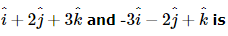Solution:
QUESTION: 25

The smallest positive integral value of P for which the equation cos (P sin x) = sin (P cos x) in x has a solution in [0, 2π] is

Solution:
QUESTION: 26

Let A, B, C be three angles such that A = π/4 and tan B tan C = p. Then all possible values of p such that A, B, C are the angles of a triangle is:

Solution:
QUESTION: 27

If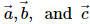are non-coplanar unit vectors such that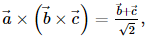, then the angle between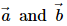is

Solution:
QUESTION: 28

In the following question, a Statement of Assertion (A) is given followed by a corresponding Reason (R) just below it. Read the Statements carefully and mark the correct answer-
Assertion(A): The term independent of x in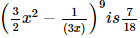Reason(R): The number of terms in the expansion of (x+y+z)n, where n is a +ve integer, is 1/2 (n + 1) (n + 2).

Solution:
QUESTION: 29

In the following question, a Statement of Assertion (A) is given followed by a corresponding Reason (R) just below it. Read the Statements carefully and mark the correct answer-
Assertion(A): The number of diagonals that can be drawn in a plane figure of 16 sides is 120 .
Reason(R): Number of circular arrangements of n different things = (n + 1)!.

Solution:
QUESTION: 30

In the following question, a Statement of Assertion (A) is given followed by a corresponding Reason (R) just below it. Read the Statements carefully and mark the correct answer-
Assertion(A): Tangent to the curve y2 = 2x3 which is perpendicular to the line 4x - 3y + 2 = 0 is drawn at the point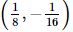Reason(R): m1.m2 = -1 , if two lines are perpendicular.

Solution: